## Available courses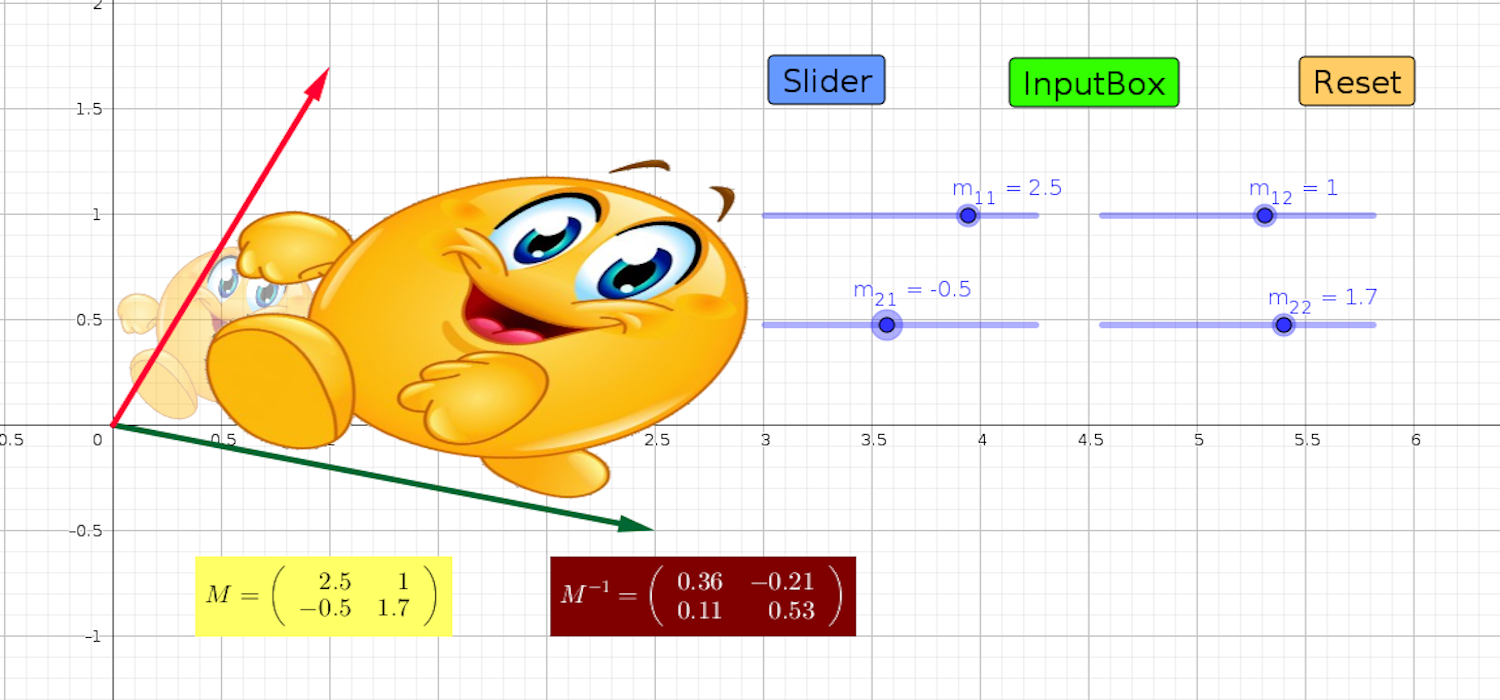#### Introductory Linear Algebra & Analytical Geometry

Linear algebra is concerned with the study of vectors, vector spaces, and linear transformations between vector spaces. Linear algebra has among its many applications a basic connection with coordinate geometry. For instance, lines, planes, and conic sections may be analysed this way.

In this course, the geometric interpretation of vectors and the operations on and between them (e.g., the scalar and vector products) is emphasized. Geometrical properties of lines and planes, possible intersection points and distances are explored. We also look at conic sections, and transformations of geometrical objects giving a geometrical interpretation of the determinant of a matrix.

Matrices also appear in the study of systems of linear equations which are solved by Gaussian elimination or Cramer’s rule. Matrix operations as well as the recursive definition of determinants are explained.

As a by-product, we also study the geometry of complex number.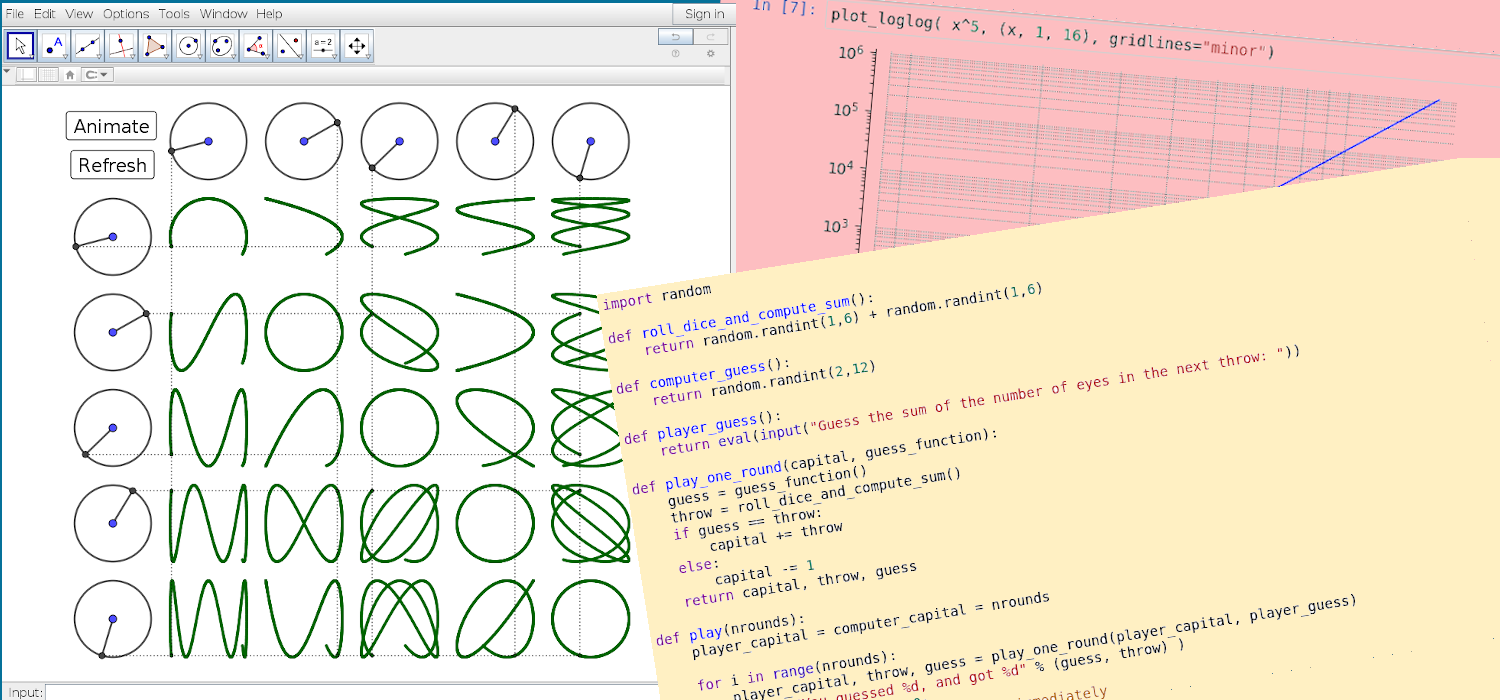#### Python Programming & Mathematical Software

The availability of computer algebra systems has been changing the mathematical landscape considerably in the past few years: Real-world problems that were deemed computationally too long-winded or complicated can now be tackled. Appropriate visualizations help interpret the results obtained, mathematical software can be used to “experiment” with mathematical structures and find connections between them.

In this course, students are provided with hands-on access to mathematics. They are introduced to the modern mathematical software package Sage and the statistical software package R via the cloud-service “CoCalc”, as well as the intuitive geometry program GeoGebra.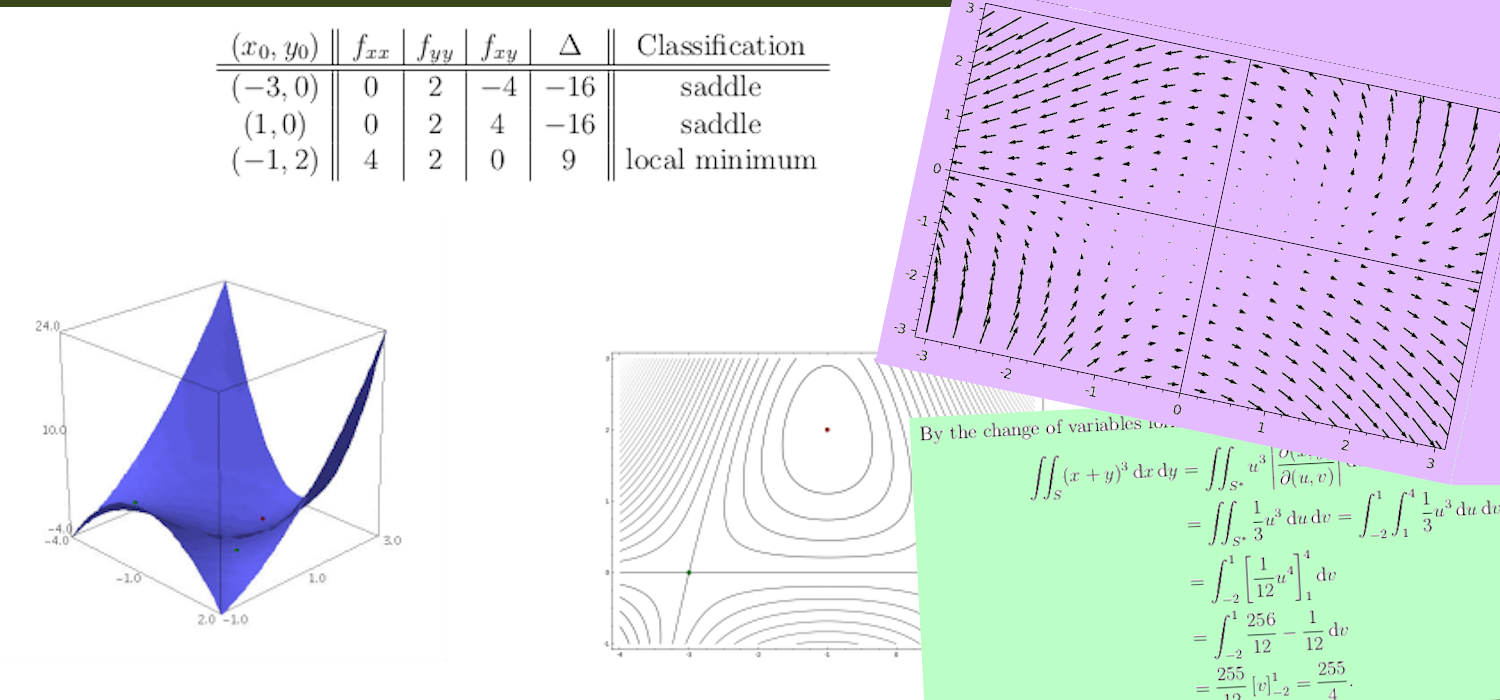#### Multivariable Calculus

Multivariable calculus (also known as multivariate calculus) is the extension of calculus in one variable to calculus with functions of several variables: the differentiation and integration of functions involving multiple variables, rather than just one. Techniques of multivariable calculus are used to study many objects of interest in the material world like curves, surfaces and vector fields.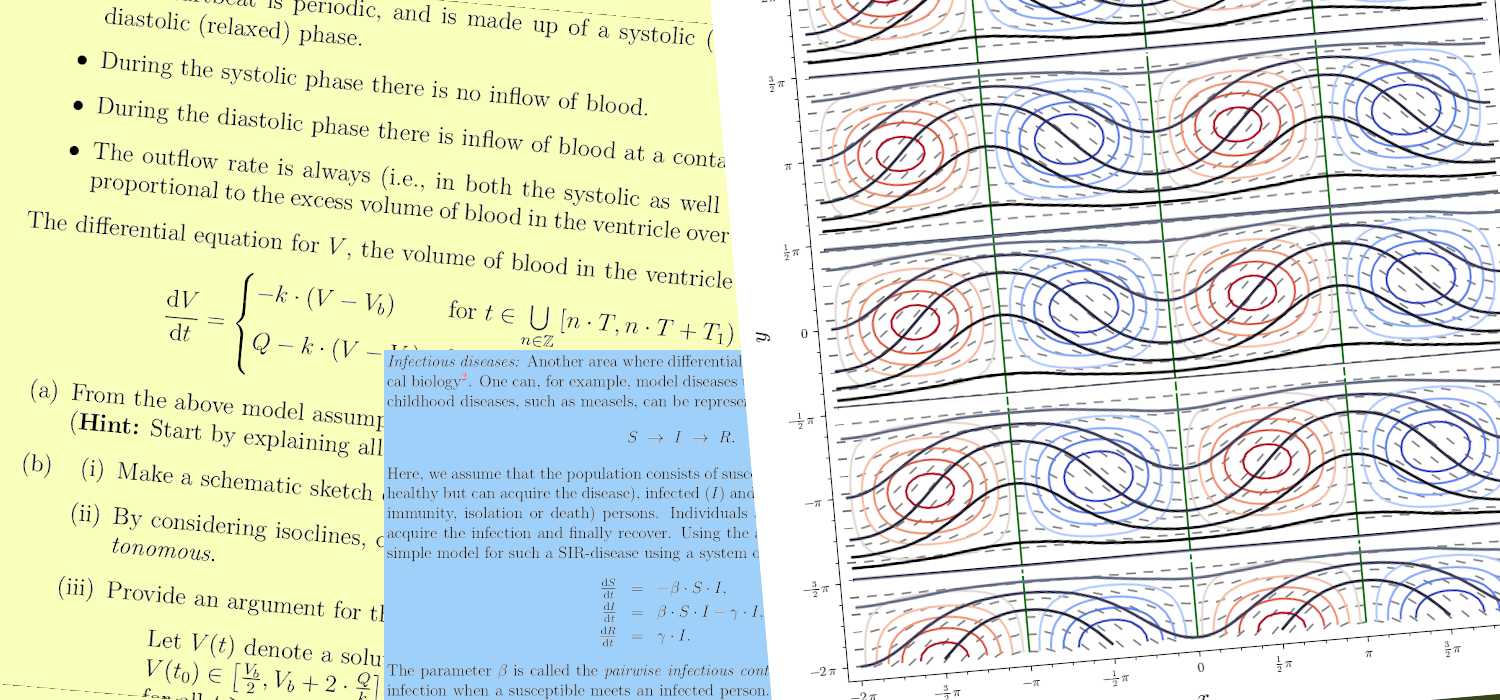#### Differential Equations

Ordinary differential equations (ODEs) arise in many contexts of mathematics and science (social as well as natural). Mathematical descriptions of change use differentials and derivatives. Various differentials, derivatives, and functions become related to each other via equations, and thus a differential equation is a result that describes dynamically changing phenomena, evolution, and variation. Often, quantities are defined as the rate of change of other quantities (for example, derivatives of displacement with respect to time), or gradients of quantities, which is how they enter differential equations.#### Abstract Algebra 2

This course continues, complements and deepens the study of algebraic ideas and concepts as begun in MATH2310 Abstract Algebra 1 (and thus these two courses are offered in consecutive semesters). Structures like groups, rings, and fields as well as mappings like homomorphisms and isomorphisms will be revisited in this course.

In this course, students will explore groups in greater depth; in particular, group actions are used to prove several key results about finite groups, and the famous Sylow Theorems are discussed. Students will be exposed to certain aspects used in the classification of finite simple groups, like the Decomposition Theorem and the Jordan-Hölder Theorem.

The course then progresses to topics in algebraic number theory where students learn to construct algebraic extensions containing the root of a polynomial. Several interesting and motivating examples like the straigthedge and compass constructions will be discussed.#### Real Analysis 2

This course exposes students to rigorous mathematical definitions of derivatives and (Riemann) integrals, together with topics like uniform convergence of a sequence of functions and series of functions. It continues and completes the study of the rigorous foundations of calculus begun in MATH2321 Real Analysis 1. A major emphasis is placed on the proper use of definitions for the rigorous proof of theorems.

This course is compulsory for all students pursuing a major in mathematics. Students interested in advanced analytical courses like complex analysis, Fourier analysis, numerical analysis will need a good grasp of the concepts discussed in this course.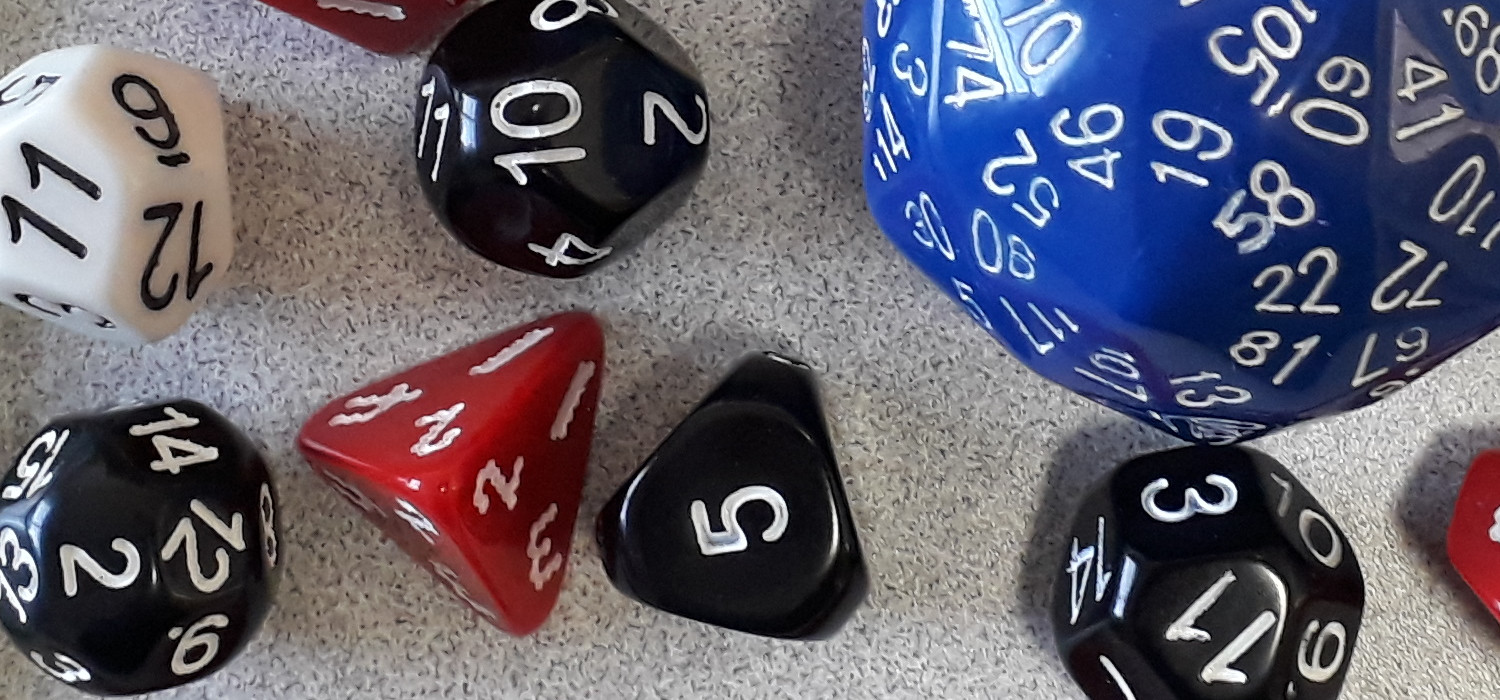#### Probability Theory 1

This course begins with a discussion of the basic ideas of probability, including the axioms of probability, combinatorial probability, conditional probability and independence. The rest of the course focuses on distribution theory. The distribution theory of one discrete and one continuous random variable is discussed. Special attention is paid to well-known discrete and continuous distributions such as the Bernoulli, Binomial, Poisson, Exponential, Gamma and Normal. Also, the distribution theory of several random variables is discussed.

The idea of a statistic is introduced and the distribution theory of the mean and the sample variance is described. This leads finally to the idea of convergence in distribution and the Central Limit Theorem (without proof). Overall, the approach taken is non-rigorous. In particular, there will be no mention of sigma algebras or of measure theory.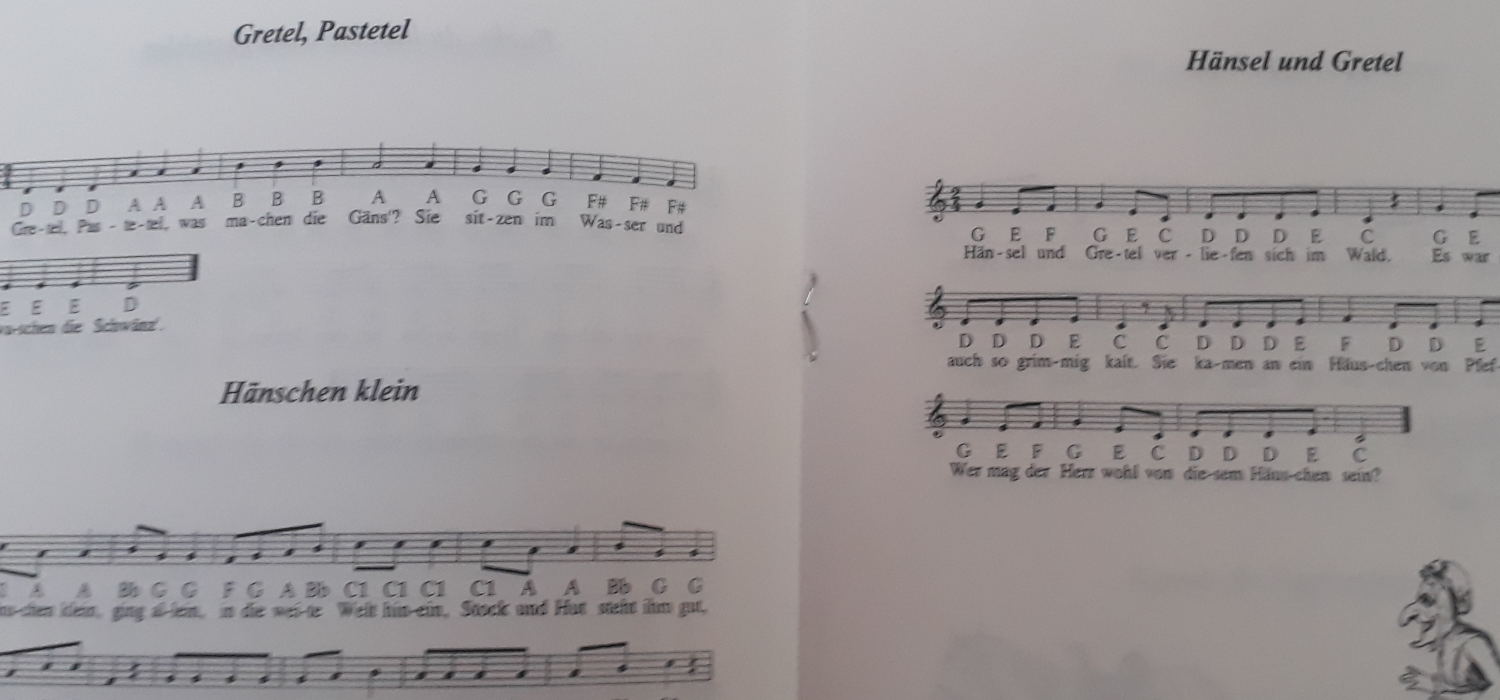#### Composition

Composition or Compositions may refer to:
• Composition (language), in literature and rhetoric, producing a work in spoken tradition and written discourse, to include visuals and digital space
• Composition (music), an original piece of music and its creation
• Composition (visual arts), the plan, placement or arrangement of the elements of art in a work
• Composition (dance), practice and teaching of choreography
• Function composition (mathematics), an operation that takes functions and gives a single function as the result
• Composition of relations (mathematics), an operation that takes relations and gives a single relation as the result
• Composition (combinatorics), a way of writing a positive integer as a sum of positive integers
• Composition algebra (mathematics), an algebra over a field with composing norm
• Binary function or law of composition